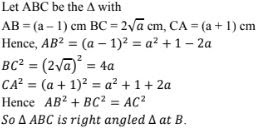# RD Sharma Solutions Chapter 4 Triangles Exercise 4.7 Class 10 Maths

 Chapter Name RD Sharma Chapter 4 Triangles Book Name RD Sharma Mathematics for Class 10 Other Exercises Exercise 4.1Exercise 4.2Exercise 4.3Exercise 4.4Exercise 4.5Exercise 4.6 Related Study NCERT Solutions for Class 10 Maths

### Exercise 4.7 Solutions

1. If the sides of a triangle are 3 cm, 4cm, and 6cm along, determine whether the triangle is a right - angled triangle.

Solution

We have,
Sides of triangle
AB = 3cm
BC = 4 cm
AC = 6cm
∴ AB2 = 32 = 9
BC2 = 42 = 16
AC2 = 62 = 36
Since, AB2 + BC2 ≠ AC2
Then, by converse of Pythagoras theorem, triangle is not a right triangle.

2. The sides of certain triangles are given below. Determine which of them right triangles are.
(i) a = 7 cm, b = 24cm and c = 25cm
(ii) a = 9cm, b = 16 cm and c = 18cm
(iii) a = 1.6cm, b = 3.8cm and c = 4cm
(iv) a = 8cm, b = 10cm and c = 6 cm

Solution

We have,
a = 7cm, b = 24 cm and c = 25cm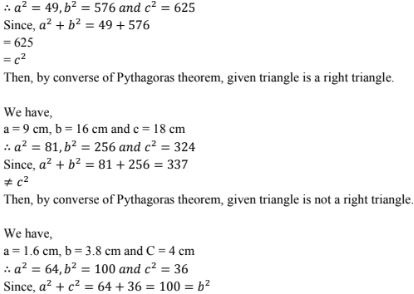Then, by converse of Pythagoras theorem, given triangle is a right triangle.

3. A man goes 15 metres due west and then 8 metres due north. How far is he from the starting point ?

Solution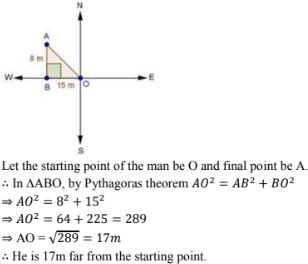4. A ladder 17m long reaches a window of a building 15m above the ground. Find he distance of the foot of the ladder from the building.
Solution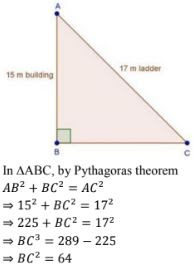⇒ BC = 8m
∴ Distance of the foot of the ladder from building  = 8m

5. Two poles of heights 6m and  11m stand on a plane ground. If the distance between their feet is 12m, find the distance between their tops.
Solution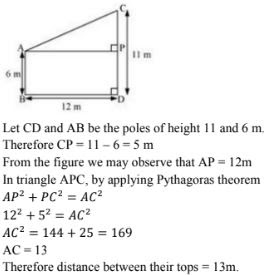6. In an isosceles triangle ABC, AB = AC = 25 cm, BC = 14cm. Calculate the altitude from A on BC.
Solution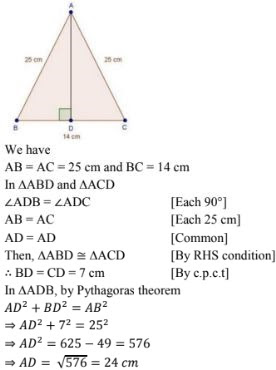7. The foot of a ladder is 6m away from a wall and its top reaches a window 8m above the ground. If the ladder is shifted in such a way that its foot is 8m away from the wall, to what height does its tip reach ?
Solution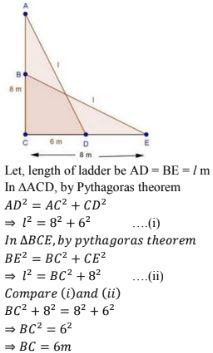8. Two poles of height 9m and 14m stand on a plane ground. If the distance between their feet is 12m, find the distance between their tops.

Solution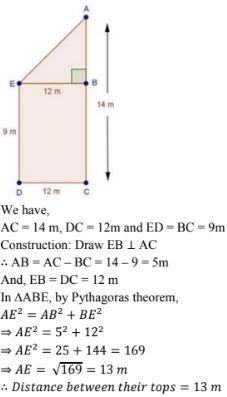9. Using Pythagoras theorem determine the length of AD in terms of b and c shown in Fig. 4.219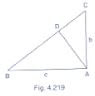Solution

We have,
In ΔBAC, by Pythagoras theorem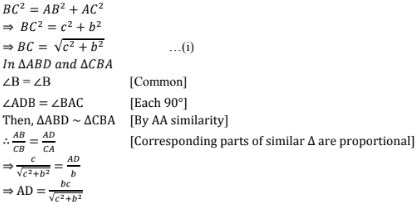10.  A triangle has sides 5cm, 12cm and 13cm. Find the length to one decimal place, of the perpendicular from the opposite vertex to the side whose length is 13 cm.
Solution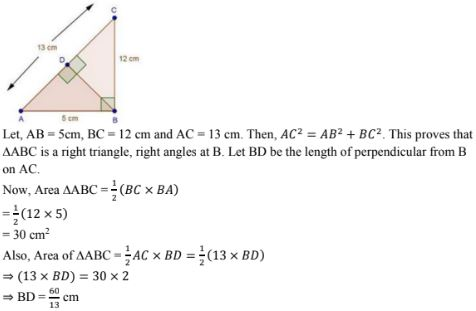11. ABCD is a square. F is the mid - point of AB. BE is one third of BC. If the area of ΔFBE = 108 cm2 , find the length of AC.
Solution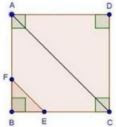Since, ABCD is a square
Then, AB = BC = CD = DA = x cm
Since, F is the mid - point of AB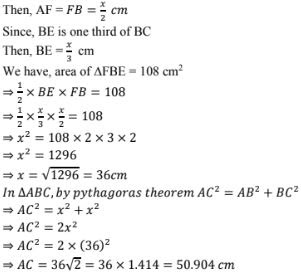12. In an isosceles triangle ABC, if AB = AC = 13 cm and the altitude from A on BC is 5cm, find BC.
Solution∴ BD = CD = 12 cm  [By c.p.c.t]
Hence, BC = 12 + 12  = 24 cm

13. In a ΔABC, AB = BC = CA = 2a and AD ⊥ BC. Prove that
(ii) Area(ΔABC) = √3a2
Solution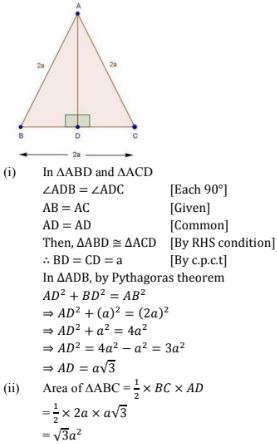14. The lengths of the diagonals of a rhombus are 24 cm and 10cm. Find each side of the rhombus.
Solution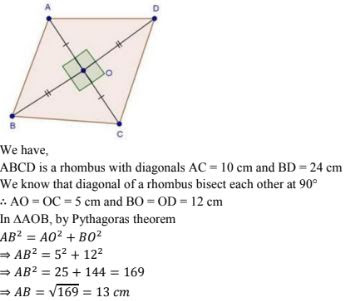15. Each side of a rhombus is 10 cm. If one of its diagonals is 16cm find the length of the other diagonal.
Solution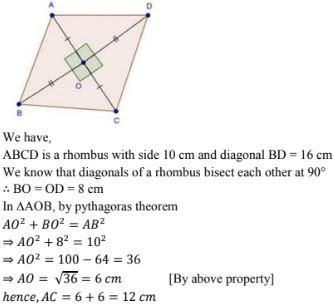16. In an acute - angled triangle, express a median in terms of its sides.
Solution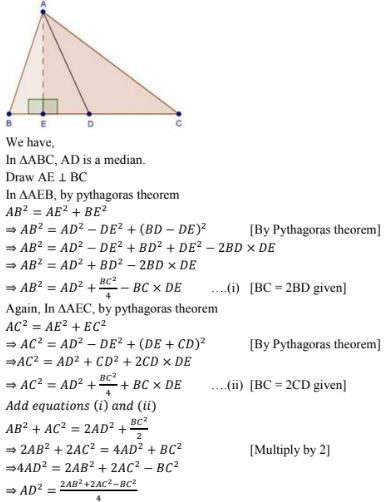17. Calculate the height of an equilateral triangle ach of whose sides measures 12 cm.
Solution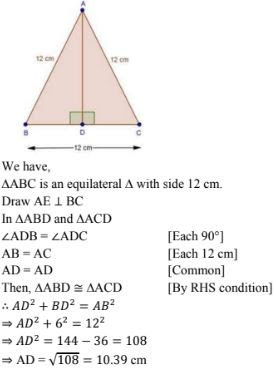18. In right - angled triangle ABC in which ∠C = 90°, if D is the mid - point of BC, prove that AB2 = 4 AD2 - 3AC2 .
Solution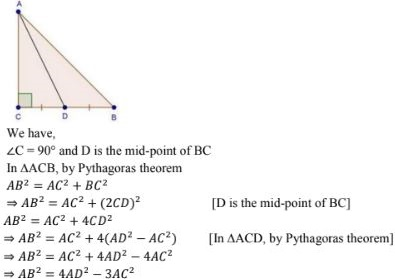19. In Fig.  4.220, D is the mid - point of side BC and AE ⊥ BC. If BC = a, AC = b, AB = c, ED = x, AD = p and AE = h, prove that :
Solution
We have, D as the mid - point of BC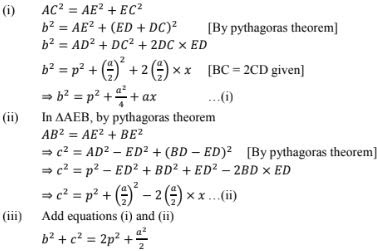20. In Fig., 4.221, ∠B < 90° and segment AD ⊥ BC, show that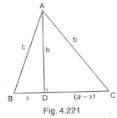(i) b2 = h2 + a2  + x2  - 2ax
(ii)  b2 = a2 + c2  - 2ax

Solution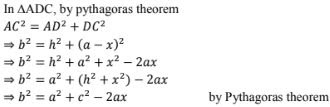21. In ΔABC, ∠A is obtuse, PB ⊥ AC and QC ⊥ AB. Prove that:
(i) AB × AQ = AC × AP
(ii) BC2  = (AC × CP  + AB × BQ)
Solution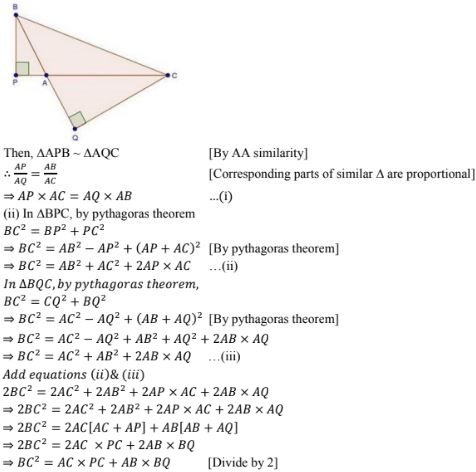22. In a right ΔABC right - angled at C, if D is the mid - point f BC, prove that BC2  = 4(AD2  - AC2)
Solution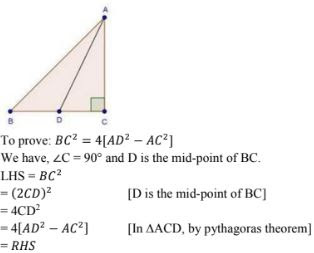23. In a quadrilateral ABCD, ∠B = 90° , AD2  =  AB2  + BC2  + CD2  , prove that ∠ACD = 90° .
Solution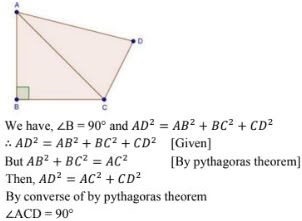24. In an equilateral ΔABC, AD⊥ BC,  prove that AD2  = 3BD2 .
Solution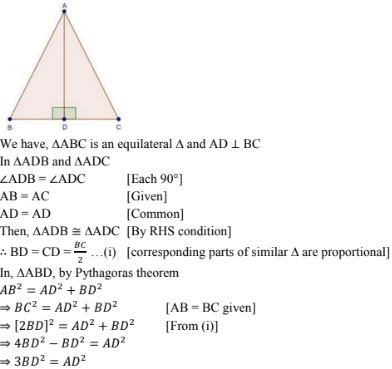25. ΔABD is a right triangle right angled at A and AC ⊥ BD. Show that :
(i) AB2 = CB × BD
(ii) AC2 = DC × BC
(iii) AD2 = BD × CD
(iv) AB2/AC2 = BD/DC
Solution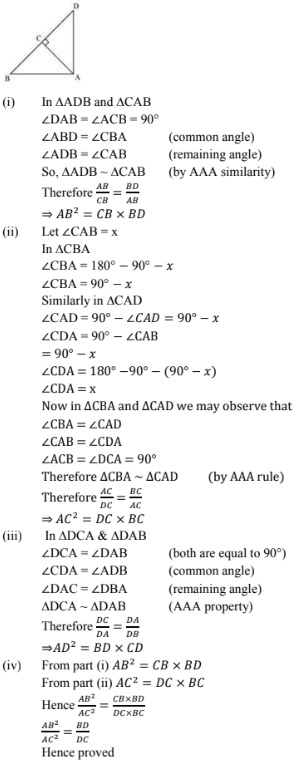26. A guy wire attached to a vertical pole of height 18m is 24m long and has  a stake attached to the other end. How far from the base of the pole should the stake be driven so that the wire will be taut ?
Solution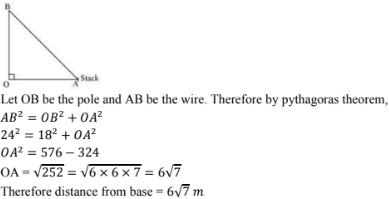27. An aeroplane leaves an airport and flies due north at a speed of 1000 km/hr. At the same time, another aeroplane leaves the same airport and flies due west at a speed of 1200 km/hr. How far apart will be the two planes after 1 hours ?
Solution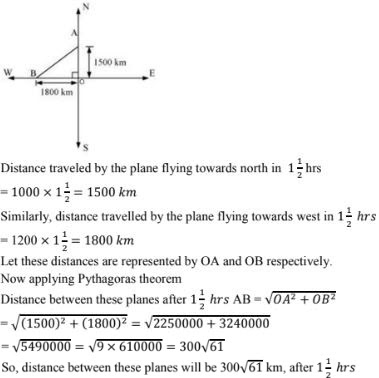28. Determine whether the triangle having sides (a - 1 )cm, 2√a cm and (a + 1) cm is a right - angled triangle.
Solution